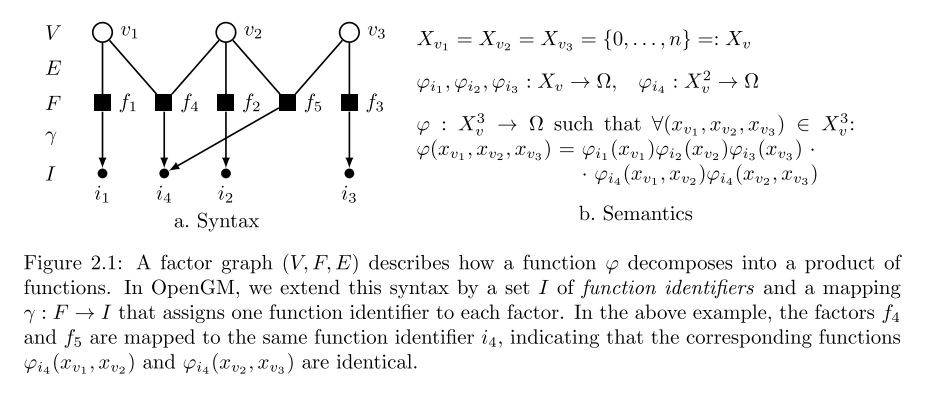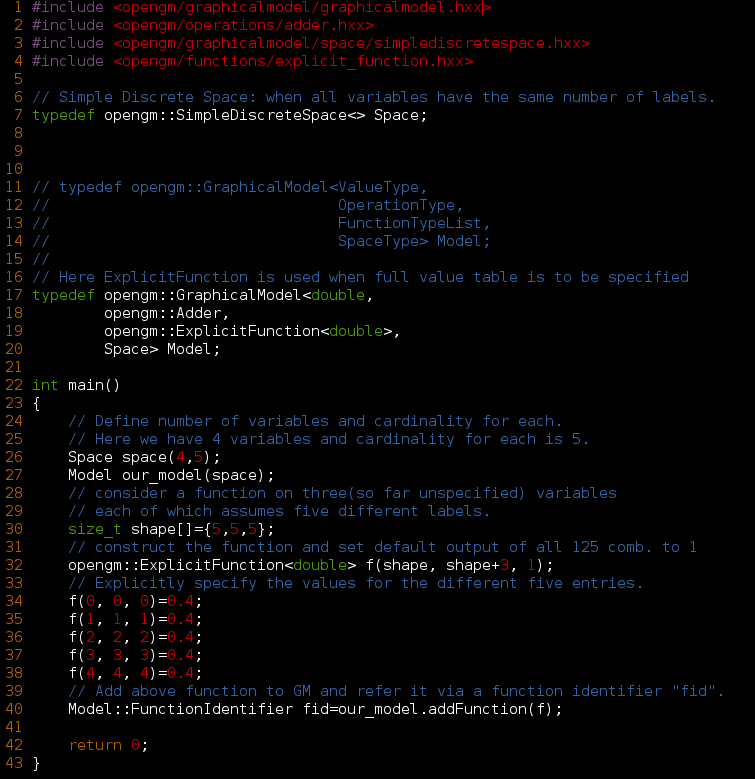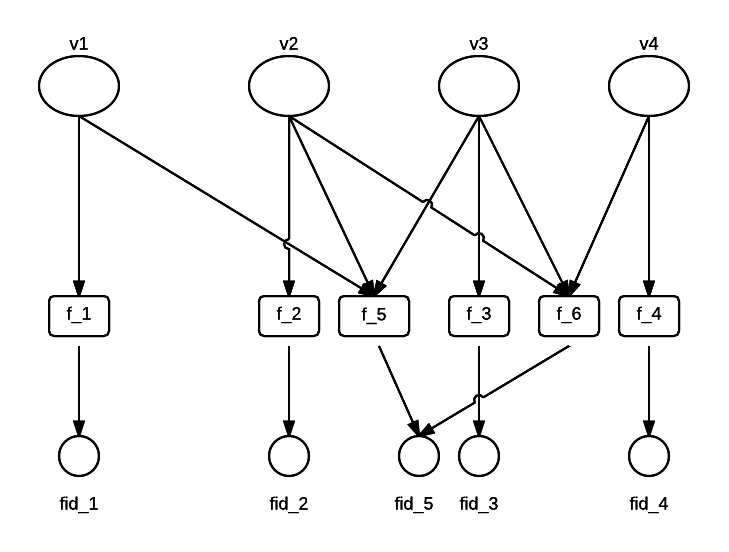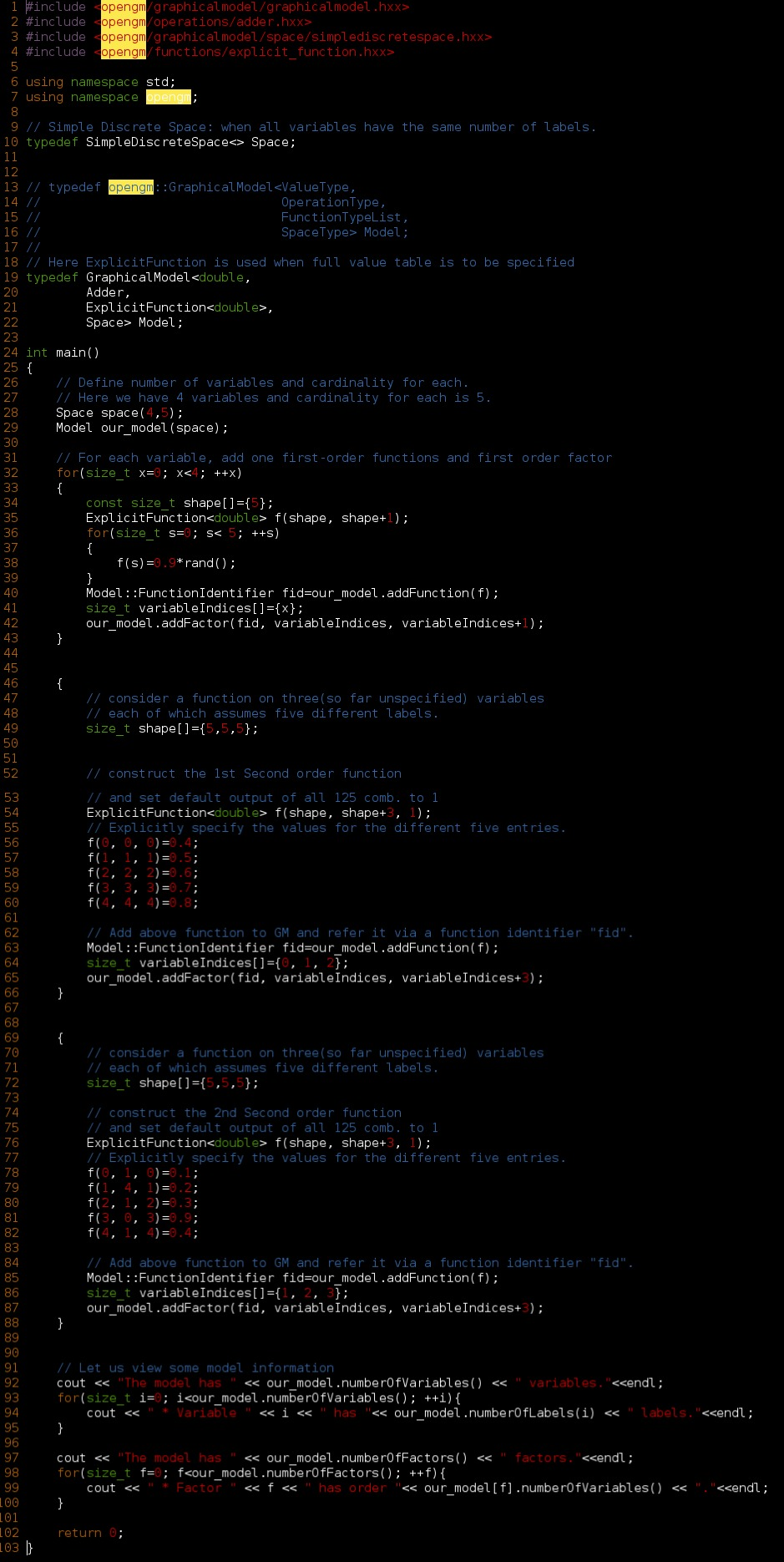I had been trying hard to get accustomed with the OpenGM library so that I get to understand what are the things that I will be needing for my implementation of Approximate algorithms.

I will jot down my findings here:

Below is the most important image that will help me to write off things quite cleanly.The things that are needed by the OpenGM for completely specifying the above factor model are:

1. The number of variables along with cardinality (the number of labels) of each of these. This is done by constructing an object called an Label Space.
2. Functions describe the different small $latex \varphi_i$ that are used to decompose the bigger $latex \varphi$.
3. Operations can be addition, multiplication, max and min. These are the operations through which the $latex \varphi$ decomposes into $latex \varphi_i$.
4. Factors is the only missing link which connects the appropriate $latex \varphi$ with respective $latex x_v's$ intended as the inputs.

Writing a complete model in OpenGM using ExplicitFunctions.The above one was a very primitive model. Let us make from a complete one like present below: